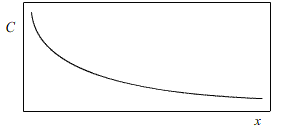# 20.2: Fick's Second Law of Diffusion

$$\newcommand{\vecs}{\overset { \rightharpoonup} {\mathbf{#1}} }$$ $$\newcommand{\vecd}{\overset{-\!-\!\rightharpoonup}{\vphantom{a}\smash {#1}}}$$$$\newcommand{\id}{\mathrm{id}}$$ $$\newcommand{\Span}{\mathrm{span}}$$ $$\newcommand{\kernel}{\mathrm{null}\,}$$ $$\newcommand{\range}{\mathrm{range}\,}$$ $$\newcommand{\RealPart}{\mathrm{Re}}$$ $$\newcommand{\ImaginaryPart}{\mathrm{Im}}$$ $$\newcommand{\Argument}{\mathrm{Arg}}$$ $$\newcommand{\norm}{\| #1 \|}$$ $$\newcommand{\inner}{\langle #1, #2 \rangle}$$ $$\newcommand{\Span}{\mathrm{span}}$$ $$\newcommand{\id}{\mathrm{id}}$$ $$\newcommand{\Span}{\mathrm{span}}$$ $$\newcommand{\kernel}{\mathrm{null}\,}$$ $$\newcommand{\range}{\mathrm{range}\,}$$ $$\newcommand{\RealPart}{\mathrm{Re}}$$ $$\newcommand{\ImaginaryPart}{\mathrm{Im}}$$ $$\newcommand{\Argument}{\mathrm{Arg}}$$ $$\newcommand{\norm}{\| #1 \|}$$ $$\newcommand{\inner}{\langle #1, #2 \rangle}$$ $$\newcommand{\Span}{\mathrm{span}}$$$$\newcommand{\AA}{\unicode[.8,0]{x212B}}$$

Fick’s second law is concerned with concentration gradient changes with time.By considering Fick’s 1st law and the flux through two arbitrary points in the material it is possible to derive Fick’s 2nd law.

$\dfrac{\partial C}{\partial t} = D\left( \dfrac{\partial ^2C}{\partial x^2} \right)$

This equation can be solved for certain boundary conditions:

## 1. “Thin source”

Consider a semi-infinite bar with a small, fixed amount of solute material diffusing in from one end.The amount of solute in the system must remain constant, therefore

$\int \limits_0^\infty C\left( {x,t} \right ) \,dx = B$

where $$B$$ is a constant.

The initial concentration of solute in the bar is zero, therefore

$C\left( x,t = 0 \right ) = C_0$

These boundary conditions give the following solution:

$C\left( x,t \right ) = \frac{B}{\sqrt {\pi Dt}}\exp \left( \frac{ - x^2}{4Dt} \right )$

## 2. “Infinite source”

A semi-infinite bar with a constant source (i.e. constant concentration) of solute material diffusing in from one end.In this case, the solution is obtained by stacking a series of “thin sources” at one end of the bar, and summing the effects of all of the sources over the whole bar.

The initial concentration of solute in the bar is $$C_0$$, therefore

$C\left( {x,t = 0} \right ) = {C_0}$

The concentration of solute at the end of the bar is a constant, $$C_s$$, therefore

$C\left( {x = 0,t} \right ) = {C_s}$

These boundary conditions give the following solution:

$C\left( x,t \right ) = C_s - (C_s - C_0) erf \left( \frac{x}{2\sqrt {Dt}} \right )$

erf{x} is known as the error function and results from the summation of the thin sources at the end of the bar. It is defined as

$erf\left( x \right ) = \frac{2}{\sqrt \pi } \int \limits_0^x \exp ( - u^2 ) du$

The integral can only be solved numerically with a computer, so erf tables are used to solve the diffusion equation where necessary.

This animation shows the applications of Fick’s 2nd law and its solutions.

## 3. Non-Analytical Solutions

For more complicated situations we cannot obtain an analytical solution for Fick’s 2nd law. In these cases numerical analysis is used. Solutions obtained in this way are approximations, however, they can be made as precise as needed.

The following demonstration shows how numerical analysis can be used to approximate solutions for various conditions.